## Red-Black Tree Tutorial

### things behind the tricks

Red-Black Tree is tricky. If you have learned about Red-Black Tree, please forget what you have learned temporarily, follow this tutorial, then go back to your Red-Black textbook. You'll find the RBtree is not that difficult to grasp.

## Prerequisites

Before reading this tutorial, you should have known:

• Binary Search Tree

• 2-3-4 Tree

If you don't know what 2-3-4 tree is, don't panic. Search it and study it right now. The concept of 2-3-4 tree is simple. I think you can understand the idea in a few minutes. Understanding the idea will be enough. You don't have to write any code to implement a 2-3-4 tree.

## 2-3-4 Tree, transform!

2-3-4 tree is good, but it is not binary. We are fond of binary trees. Can we represent a 2-3-4 tree in a binary way? Obama has told you: yes, we can.

Let's study the 3 kinds of node in a 2-3-4 tree.

2-node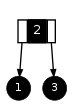, apparently, is already binary. We can map a 2-node directly to a binary tree node: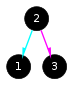(We use different colors to denote arrows pointing to different children, cyan for left and magenta for right. You'll find the coloring useful after studying trees for 10 hours and try to point out which one is left subtree and which one is right).

3-node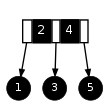is a little more complicated, but not difficult. We can represent a 3-node in two different ways: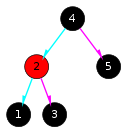and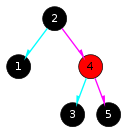. From this moment on, you will see different colors in a binary tree nodes: black and red. When you see a red node, say N, please remember N and parent(N) are supposed to live in the same node. It's very important to keep this in your mind. Once you find yourself confused by any Red-Black Tree operation, tell yourself: RBtree is simple, absorb all red nodes into their parents, they are just 2-3-4 trees!

OK, let's look at 4-node now. It's more complicated than 3-node, but not difficult either. There are 5 ways to represent a 4-node like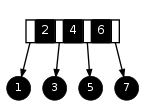: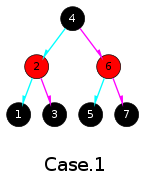,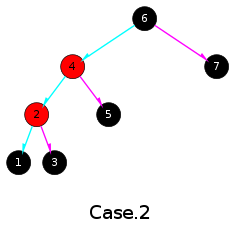,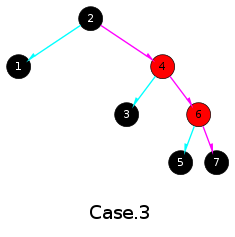,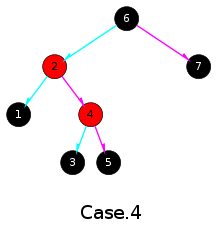,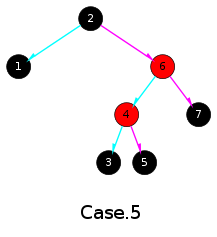. For convenience, I will call this five cases "The Gang of Five". Note that all of them have the property that the inorder traversal is ordered.

If you have learned RBtree from any other materials, you are probably told that a red node cannot have a red child, but the fact is it can. Yes it can. The only reason for us to avoid two red nodes in a row is to simplify the rules. Again, please do remember that RBtrees are essentially 2-3-4 trees. As long as a RBtree is essentially a valid 2-3-4 tree, it is a good RBtree.

## Make a decision

We listed 5 possibilities to represent a 4-node. Now have a break. Make a cup of coffee and ask yourself some questions, "why are we here? what are we doing?" The answer would be "we are trying to map 2-3-4 trees to binary trees." But why bother? Why do we learn 2-3-4 trees and try to implement one using whatever method? Ah, yes, because 2-3-4 trees are balanced. The cost of search/insertion/deletion of nodes are guaranteed O(lg(n)).

It is very important to keep in mind that we are working on balanced trees. Now look at The Gang of Five. Apparently Case.1 looks more balanced than others. Things begin to be tricky. Although all the 5 cases are "essentially" balanced 4-nodes, but they are "literally" binary trees, so when we do any operations on it, they ARE binary trees. Therefore, Case.1 is obviously better than other 4 cases and we will use it as the default representation of 4-node.

What about Case.2, 3, 4, 5? You must have guessed the answer. We love them, but we decide to avoid using them. And you've learned why: they are not as balanced.

Now you know why your textbook tells you that red nodes must not have red children. They can have red children, but for sake of efficiency, we better not let them.

## Rotation

Please do not hate Case.2-5. They are friendly. They are not standing in your way and doing evil. You'll find that we can make good use of them. They just cannot appear in the final RBtrees.

The good news is, we can always transform the Case.2-5 into Case.1 by "rotating" them. "Rotation" is an interesting operation. It changes a tree but keeps the relationship between nodes: LEFT(N) < N < RIGHT(N).

To rotate a tree, just imagine you are, well, rotating it. Let's take Case.2 for example. If we want to transform it into Case.1, we can imagine node 4 is a pivot. Imagine we can rotate the node 6 clockwise so that 6 and 7 will move around 4. When 6 and 7 have reached the positions like that in Case.1, we take 5 (and its subtrees, if any) away from 4 and give it to 6 and we're done. In this process, the moving part (node 6 and its right subtree) is at the right side of the stable part (node 4), so we call it Right-Rotate, denoted as RotR(6).

Similarly, we can Left-Rotate Case.3 to get Case.1. The rotation is denoted as RotL(2).

Case.4 and Case.5 are more complicated, because one rotation is not enough. For Case.4, we will have to RotL(2) to get Case.2, then RotR(6) to get Case.1. For Case.5, we RotR(6) then RotL(2).

Sometimes the rotations applied to Case.4 and Case.5 are called Double-Rotations. What they are called are not important. All we have to know is they are all disguised 4-nodes.

We will do a lot of rotations, please make sure you have learned how to perform Left-Rotations and Right-Rotations.

## Flip

2-3-4 tree has many interesting properties. Look at this 2-3-4 tree: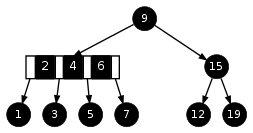, if we move 4 to its parent node, we get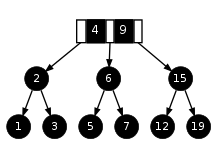. The former tree contains one 4-node, while the latter tree contains one 3-node.

Let's have a look at the counterparts of them in the Red-Black world, those would be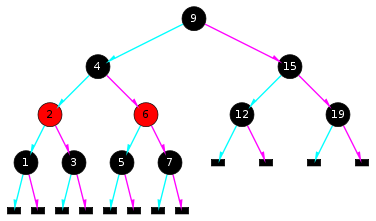and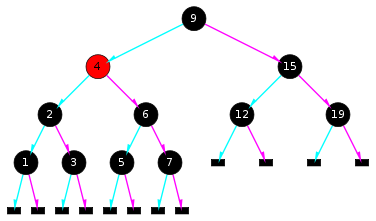. That is to say, if COLOR(N) is black and both COLOR(LEFT(N)) and COLOR(RIGHT(N)) are red, we can change the color of the three node at the same time and get another tree.Interestingly and importantly, the new tree is balanced too. We call this color-changing process "flip", since it's like fliping the colors over.

So far we have had two weapons to manipulate RBtrees: Rotation and Flip. Both of them keeps the tree balanced - I mean if we represent the RBtree as a 2-3-4 tree, it's perfectly balanced.

## Red-Black Tree

Till now, you have learned everything about Red-Black Tree. You may not believe this, because we just talked about how to represent 2-3-4 trees with Black and Red nodes. I'm telling the truth, however, because RBtrees are 2-3-4 trees.

If you doubt it, let's have a look at the definitive description of Red-Black Tree (from Wikipedia):

1. A node is either red or black.

2. The root is black. (This rule is sometimes omitted from other definitions. Since the root can always be changed from red to black but not necessarily vice-versa this rule has little effect on analysis.)

3. All leaves are black.

4. Both children of every red node are black.

5. Every simple path from a given node to any of its descendant leaves contains the same number of black nodes.

(Do not read the Red-Black Tree item in Wikipedia, it is not as good as mine :-)

Let's see why RBtrees are defined this way:

1. A node is either red or black - red nodes is combined with its parent to form a 3-node or 4-node

2. The root is black - the root has no parent, so a red root would be meaningless

3. All leaves are black - note that the "leaves" are nil nodes

4. Both children of every red node are black - we've explained this, bazinga

5. Every simple path from a given node to any of its descendant leaves contains the same number of black nodes - because number of black nodes implies the height of the 2-3-4 tree

OK, it's time to have a glance at a bigger RBtree: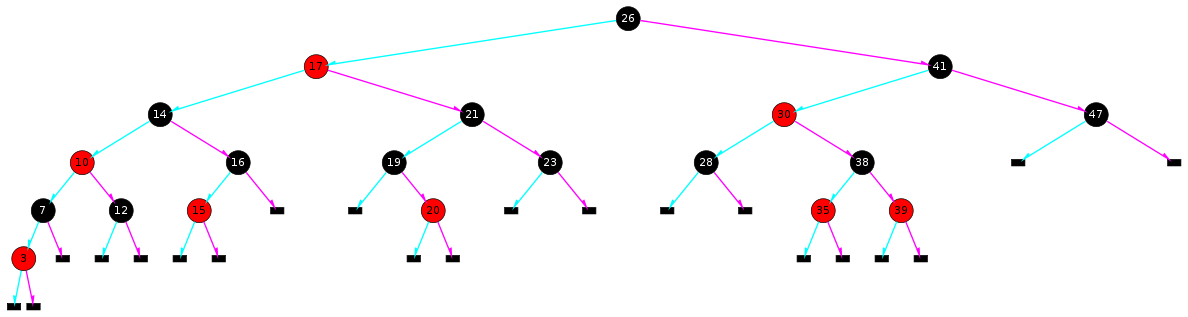Red-Black Tree

There are six 3-nodes and one 4-node in this RBtree. The 3-nodes are {3,7}, {10,14}, {15,16}, {17,26}, {19,20}, {30,41}. The 4-node is {35,38,39}.

## Search

Searching is trvial.

```NODE * rb_search(NODE * T, int v)
{
if (T == nil)
return 0;

if (v < T->val)
return rb_search(T->L, v);
else if (v > T->val)
return rb_search(T->R, v);
else
return T;
}
```

## Insert

Always remember that we are manipulating disguised 2-3-4 trees, so think in 2-3-4 trees. In 2-3-4 trees, we insert a node by putting the new value into an existing node so that a 2-node becomes a 3-node, a 3-node becomes a 4-node, and a 4-node becomes, well, an invalid node - we cannot insert anything into a 4-node. What do we do then? The answer is to avoid end up with an insertion into a 4-node by fliping (see Flip) every 4-node to split it into two 2-nodes when we go downward.

Note that the new node is always added to a leaf, therefore when we keep flipping 4-nodes, it can be guaranteed that we will not end up with a 4-node.

There are 3 kinds of nodes in a 2-3-4 tree: 2-node, 3-node and 4-node. Note that a 4-node we meet never follows a 4-node, because if it does, we would have splitted it. So a 4-node follows either a 2-node or a 3-node. We should make sure the insertion process works in both of the cases.

A 4-node following a 2-node is very simple to handle. For example: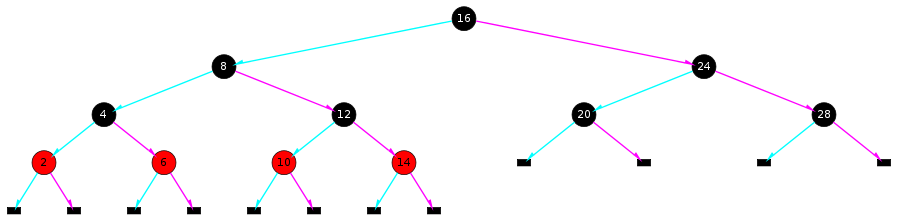Let's insert 7 into this tree. Starting from 16, we go left and left till arriving at a 4-node {2,4,6}. Then we flip: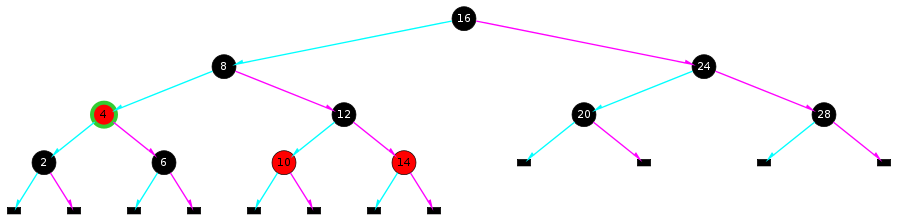Then we go right to the leaf 6 and insert 7: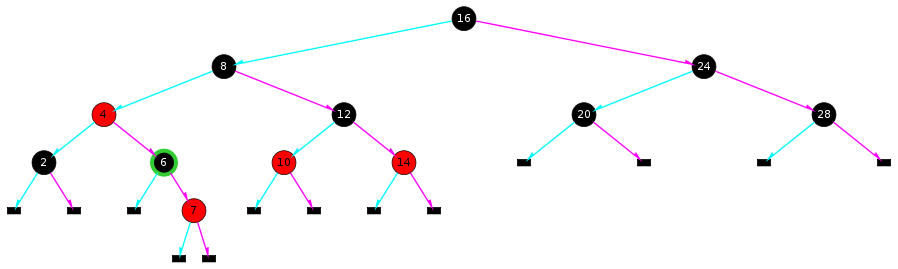A 4-node following a 3-node is a little more complicated. Look at this RBtree:

Figure 1. Red-Black Tree sample A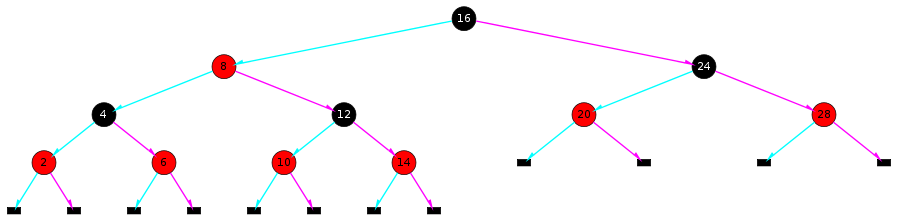This is essentially a 2-3-4 tree with a 3-node {8,16} followed by three 4-nodes {2,4,6}, {10,12,14} and {20,24,28}. Suppose we're going to insert 3. We start from 16 and find it part of a 3-node {8,16}, so we go left to 4. This is a 4-node {2,4,6}, let's split it into two 2-nodes by flipping it:

Figure 2. Red-Black Tree sample A: Flip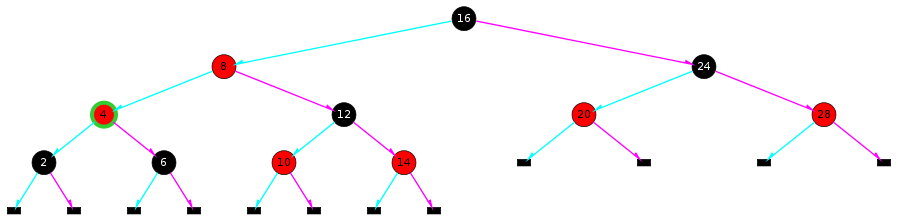Let's have a look at the new tree. {4,8,16} is a 4-node (Case.2 of The Gang of Five), which has 4 children/subtrees: 2, 6, {10,12,14} and {20,24,28}. Don't be nervous when seeing a 4-node like {4,8,16}. Take it easy, we will transform it when we go upwards.

Finally we will reach the 2-node 2 and insert 3:

Figure 3. Red-Black Tree sample A: Insert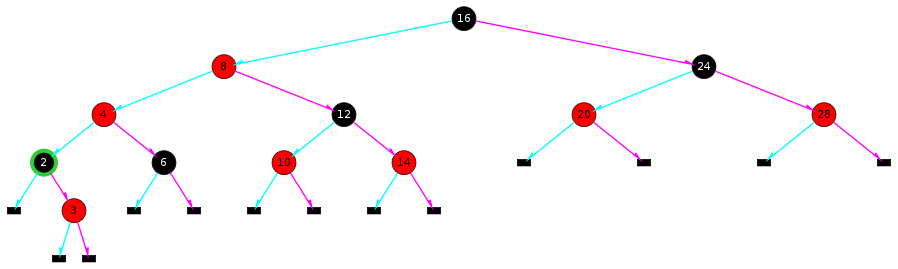The new inserted node is red because we are inserting it into an existing node, a 2-node or a 3-node.

If we allow all The Gang of Five to appear in a RBtree, we've already done. But the fact is we only accept one of them: Case.1. Therefore we must transform Case.2, Case.3, Case.4 and Case.5 into Case.1. This is the task we should do when we go upwards.

Before doing the transformation, we must recognize the four cases. Fortunately it's easy, because each of them has two red nodes in a row.

Figure 4. Red-Black Tree sample A: Before Transformation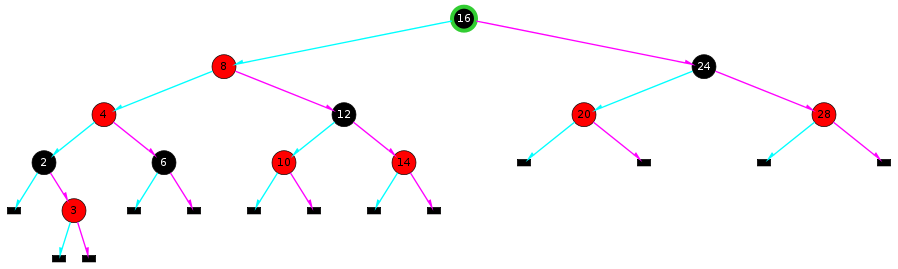This is obviously Case.2. We can perform the transformation when we reach node 16.

After transformation, we get the final RBtree:

Figure 5. Red-Black Tree sample A: After Transformation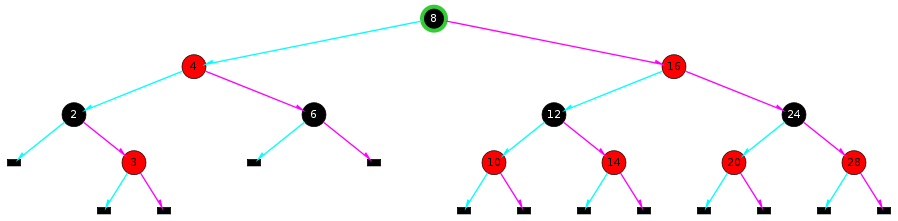The reader is urged to do Exercise 1, Exercise 2 and Exercise 3 at once.

### Implementation of Insertion

```enum RB {R, B};			/* R: Red; B: Black */

typedef struct _rbnode {
int              val;
enum RB          rb;
struct _rbnode * L;
struct _rbnode * R;
struct _rbnode * P;
} NODE;

extern NODE * nil;		/* nil points to a sentinel node with color Black */

/* REF: Robert Sedgewick, Algorithms in C */
NODE * RBinsert(NODE * h, int item, int sw)
{
int v = item;
if (h == nil)
return alloc_node(item, R);
if (h->L->rb == R && h->R->rb == R) { /* flip */
h->rb = R;
h->L->rb = B;
h->R->rb = B;
}
if (v < h->val) {
h->L = RBinsert(h->L, item, 0);
h->L->P = h;
if (h->rb == R && h->L->rb == R && sw) {  /* Case.5 */
h = rotR(h);
}
if (h->L->rb == R && h->L->L->rb == R) {  /* Case.2 */
h = rotR(h);
h->rb = B;
h->R->rb = R;
}
} else {
h->R = RBinsert(h->R, item, 1);
h->R->P = h;
if (h->rb == R && h->R->rb == R && !sw) { /* Case.4 */
h = rotL(h);
}
if (h->R->rb == R && h->R->R->rb == R) {  /* Case.3 */
h = rotL(h);
h->rb = B;
h->L->rb = R;
}
}
return h;
}

void rb_insert(int item)
{
root = RBinsert(root, item, 0);
root->rb = B;
}
```

## Deletion

We have learned a very smart insertion. It guarantees no 4-node will appear in the leaf so that we can insert without having to worry about overflow. Inspired by this, we realize that we want to reach no 2-node leaf when deleting a value, so that we don't have to worry about underflow.

The method to avoid reaching a 2-node is to combine any 2-node with part of its parent and part of its sibling so that the 2-node expands to a 3-node or 4-node. We do the combination when going downwards. We keep doing this till we find the value, if at least one of its child is nil, we just remove it; otherwise we will replace this value with its successor (the successor must satisfy the condition that at least one of its child is nil) and remove the successor.

This main idea is, like insertion, quite simple. However, to combine a 2-node with other nodes is not as straightforward as to split a 4-node. We must be very careful.

When we go downwards the tree, the only thing we know is that the parent of the current node is not a 2-node, because we just came from it. If the parent is a 2-node, we would have transformed it into a 3-node or 4-node. We don't know anything about the sibling. It can be a 2-node, or 3-node, or 4-node.

Let's assume the sibling is a 2-node. Suppose we have a 2-3-4 tree like this:

Figure 6. two 2-nodes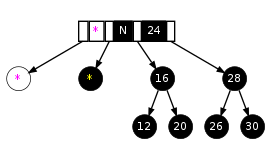The yellow asterisk represent a subtree we don't care about;

The magenta asterisks with white background mean they may or may not exist and either case has nothing to do with our following operation.

Now we want to delete 26 from it, so we will arrive at 28 and find it a 2-node. Then we'll find its sibling 16 is a 2-node too. Inspired by the flipping operation, we can just demote 24 and merge it with 16 and 28 to form a 4-node:

Figure 7. one 4-node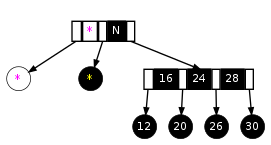If we do this in a RBtree, this process can be illustrated as from Figure 8, “Red-Black Tree sample C : two 2-nodes” to Figure 9, “Red-Black Tree sample C : one 4-node”.

Figure 8. Red-Black Tree sample C : two 2-nodes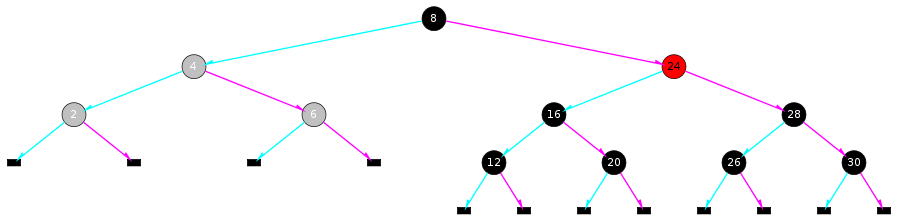Nodes 2, 4 and 6 are gray, implying that we don't care about their colors.

Figure 9. Red-Black Tree sample C : one 4-node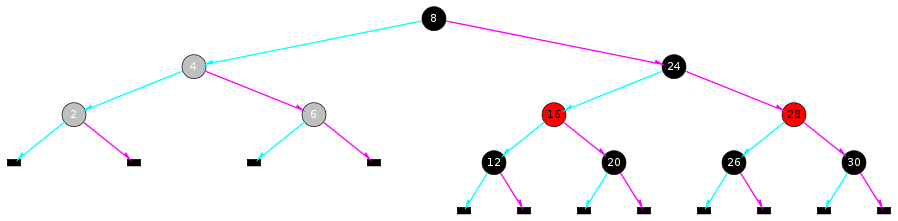In Figure 8, “Red-Black Tree sample C : two 2-nodes”, if node 4 is Red, which means {4,8,24} is a 4-node, then we have got a 3-node {4,8} followed by a 4-node {16,24,28} in Figure 9, “Red-Black Tree sample C : one 4-node”. If node 4 is Black, we have got a 2-node 8. However, if node 4 is Black, there are two different ways to represent a 3-node {8,24} (see 2-3-4 Tree, transform!). Let's see the other way to do represent Figure 8, “Red-Black Tree sample C : two 2-nodes”:

Figure 10. Red-Black Tree sample C : variant of two 2-nodes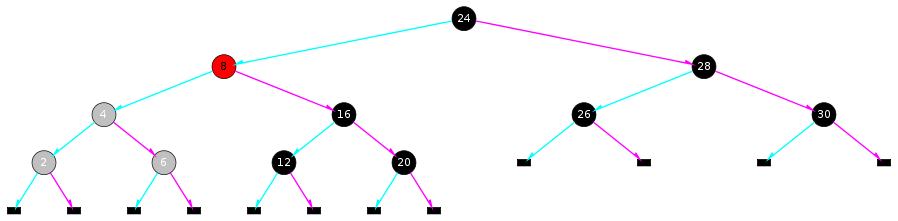This is kinda weird, since 16 and 28 are not literally siblings in this RBtree while essentially they are. So let's transform it: perform RotR(24) and we'll turn Figure 10, “Red-Black Tree sample C : variant of two 2-nodes” to Figure 8, “Red-Black Tree sample C : two 2-nodes”.

Actually we should always perform a rotation if the parent is not Red. We prefer Red because a Red node has a very useful property: its children in a RBtree representation are siblings in the corresponding 2-3-4 tree. Black nodes don't have this property. For example, in Figure 10, “Red-Black Tree sample C : variant of two 2-nodes”, the Black node 24 has two children 8 and 28, but essentially 8 is not its child, 8's children 4 and 16 are. In the meanwhile, if we see a Red node, we know for sure that its children are literally and essentially its children. You will find this property can simplify our thinking process and the operation. Therefore, from now on, we will assume the parent of the current node is Red. If it is not, we can always perform a rotation to make it Red. Don't forget that the parent is not a 2-node, so if the parent is Black, a rotation will undoubtedly make you a Red one.

OK, you've seen a 2-node sibling is quite easy to handle. What if the sibling is 3-node or 4-node? Let's see Figure 11, “a 3-node or 4-node sibling”.

Figure 11. a 3-node or 4-node sibling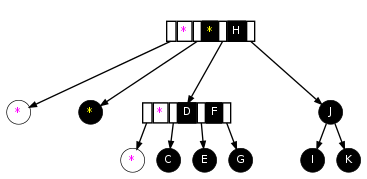Node J is a 2-node. We want to change it. Its parent is {?,H} or {?,?,H} and its sibling is {D,F} or {?,D,F}. Ah, the sibling is very crowded, why not borrowing some stuff from it? Let's borrow its rightmost part, F and the subtree rooted by G, so that the sibling becomes a 2-node {D} or 3-node {?,D}. Then we rearrange F, G and H:

Figure 12. borrowed stuff from the sibling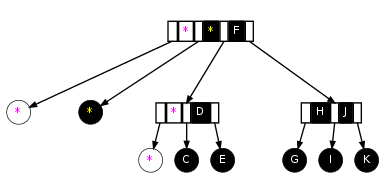This looks very good. Let's apply it to a real example in the Red-Black realm:

Figure 13. Red-Black Tree sample D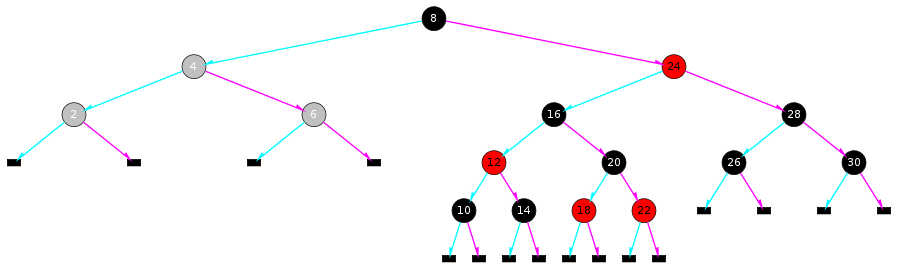Suppose we're deleting 26 from it. When we reach 28, we find it a 2-node. Then we realize its sibling is a 3-node {12,16} with 3 children: 10, 14 and {18,20,22}. Now we borrow the sibling's rightmost part, 16 and {18,20,22}, then rearrange them:

Figure 14. Red-Black Tree sample D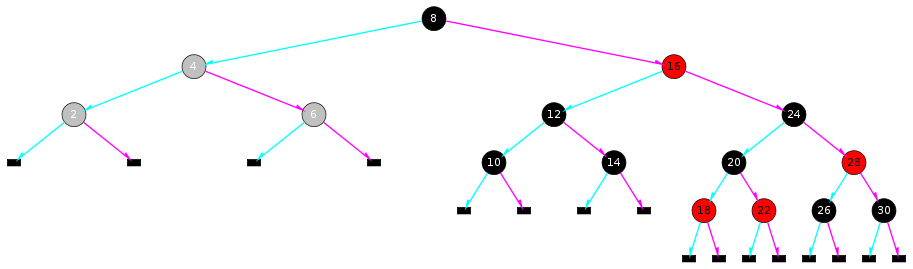As you can see, this "rearrangement" is actually a Right-Rotation with some re-coloring. No big deal, just a rotation.

Now we have a 3-node {24,28} with 3 children {18,20,22}, 26 and 30. Then we will move on. When we reach 26, we find it is a 2-node, but fortunately its sibling 30 is a 2-node too. So we flip:

Figure 15. Red-Black Tree sample D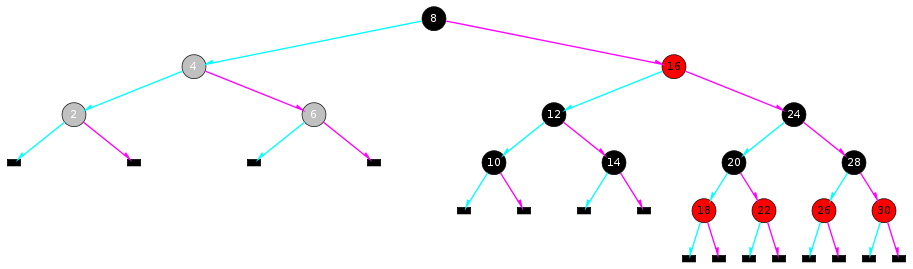Now we have a Red 26. Just delete it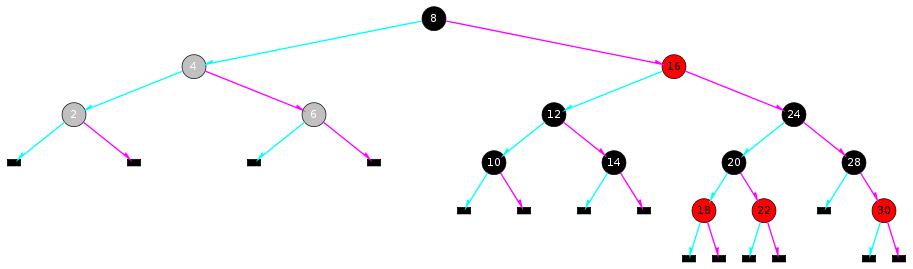and we're done.

The key point here is to borrow the rightmost part of the sibling and rearrange it. However, the position of the rightmost part of a 3-node or 4-node depends on the concrete Red-Black representation. So we must be very careful. Let's look at this example:

Figure 16. Red-Black Tree sample E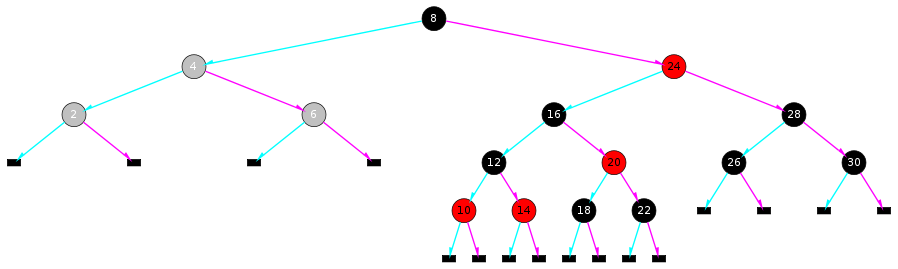In Figure 13, “Red-Black Tree sample D”, if a pointer `p` points to 28, then the rightmost part of `p`'s sibling is `p->parent->left` and the subtree rooted by `p->parent->left->right`, but here in Figure 16, “Red-Black Tree sample E”, the rightmost part would be `p->parent->left->right` and the subtree rooted by `p->parent->left->right->right`.

This complication can be solved easily. We can Left-Rotate `p->parent->left` and the situation becomes as the same as that in Figure 13, “Red-Black Tree sample D”:

Figure 17. Red-Black Tree sample E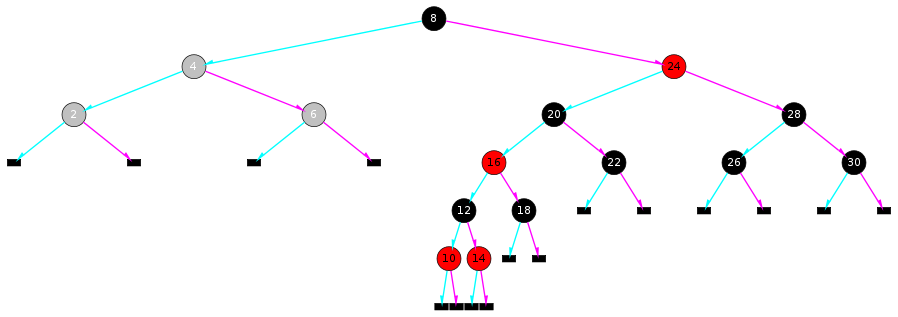Now the rightmost part of 28's sibling is 20 and 22, which are 28's parent's left child and 28's parent's left child's right subtree. You may want to compare it with Figure 13, “Red-Black Tree sample D”.

The next step will be simple for us. Perform RotR(24) and do some re-coloring:

Figure 18. Red-Black Tree sample E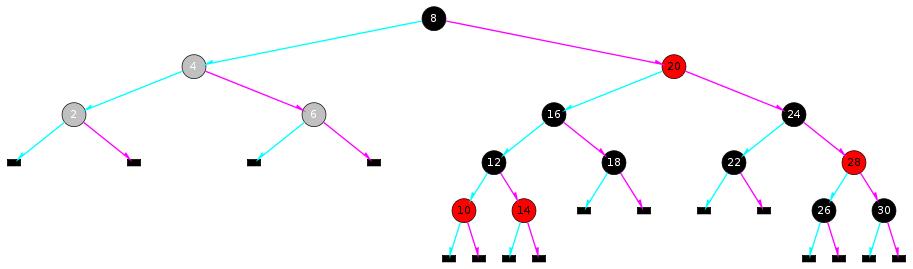Now 28 is not a 2-node anymore.

A 3-node sibling has been solved. Let's move on to see a 4-node:

Figure 19. Red-Black Tree sample F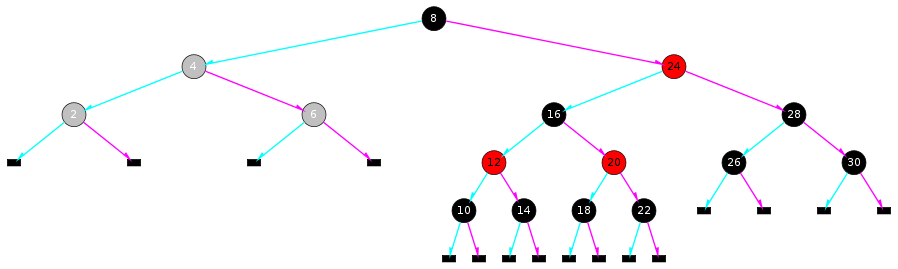Still, we want to delete 28 and it's a 2-node. Its sibling is a 4-node {12,16,20}, and the rightmost part of this 4-node is 20 and the subtree rooted by 22. This situation is quite like Figure 16, “Red-Black Tree sample E”, so let's try the same manipulation, that is, to Left-Rotation 16:

Figure 20. Red-Black Tree sample F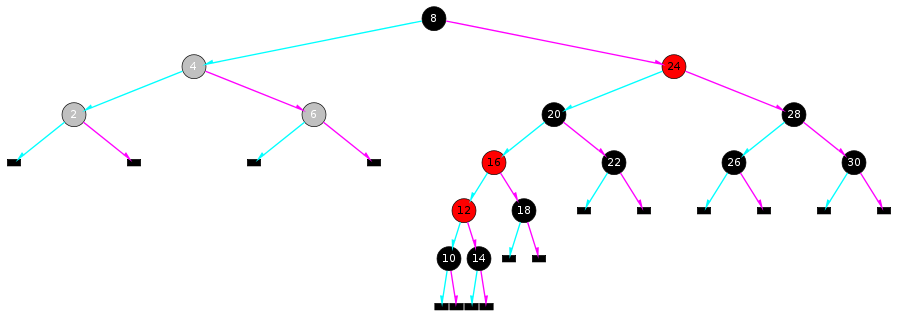There appear two Red in a row. Don't panic, it's just Case.2 of The Gang of Five! Next we do the same rotation and re-coloring as we applied to Figure 16, “Red-Black Tree sample E”:

Figure 21. Red-Black Tree sample F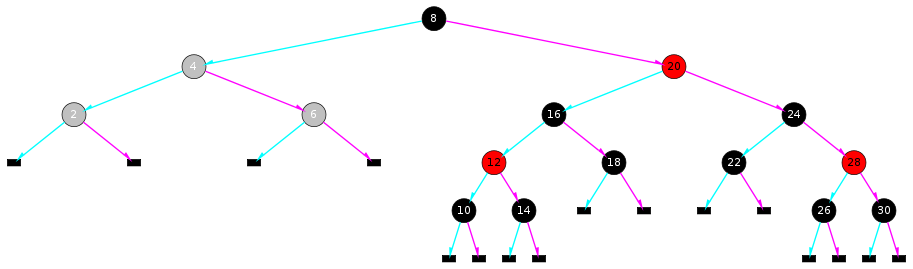Bingo! Things are done! I said we can make good use of The Gang of Five, and you see, I didn't lie.

We're almost done with all the possibilities, except one situation: what if the root is a 2-node? All the methods we have discussed use the sibling to help, but the root doesn't have any sibling. Fortunately, this can be solved easily by set the root Red as if it is a part of a 3-node or 4-node. After the deletion is finished, we can set the root back to Black (if it is still Red) without violating any RBtree rules. The reason why this works remains for you to figure out.

### Implementation of Deletion

Now you have learned all the tricks to transform a RBtree so that we won't end up with a 2-node leaf. It time to code.

```#define hL		(h->L)
#define hR		(h->R)
#define hLL		(h->L->L)
#define hLR		(h->L->R)
#define hRL		(h->R->L)
#define hRR		(h->R->R)
#define chg_rb(x)	do{(x)->rb = ((x)->rb == R) ? B : R;}while(0);
void flip(NODE * h)
{
assert(h != nil && hL != nil && hR != nil);
assert(h->rb == R);

/* h's left  child must be a 2-node */
assert(hL->rb == B && hLL->rb == B && hLR->rb == B);

/* h's right child must be a 2-node */
assert(hR->rb == B && hRL->rb == B && hRR->rb == B);

h->rb = B;
hL->rb = R;
hR->rb = R;
}
NODE * RBdelete(NODE * h, int v)
{
assert(h != nil);

if (h == root && hL->rb == B && hR->rb == B)
h->rb = R;

if (h->val == v) {
if (hL == nil || hR == nil) {
NODE * x;
if (hL != nil)
x = hL;
else
x = hR;
if (x != nil)
x->P = h->P;
if (h->P == nil) {
assert(h == root->L);
return nil;
} else if (h == h->P->L)
h->P->L = x;
else
h->P->R = x;

if (h->rb == B) {
assert(x->rb == R);
x->rb = B;
}
free_node(h);
return x;
} else {
NODE * x;
for (x = hR; x->L != nil; x = x->L) {}
h->val = x->val;
v = x->val;
}
}

/* It's possible to get here even if the ``v'' has been found:
*         when (h has two children) and (SUCCESSOR(h)->val == v).
* Therefore, we'd use (h->val <= v), not (h->val < v).
*/
if (h->val <= v) {
assert(hR != nil);

/* transform if the right child is a 2-node */
if (hR->rb == B && hRL->rb == B && hRR->rb == B) {
if (h->rb == B) {
assert(hL->rb == R);
h = rotR(h);
chg_rb(h);
chg_rb(hR);
} else {
assert(h->rb == R);
if (hL->rb == B && hLL->rb == B && hLR->rb == B) {
flip(h);
} else {
if (hLR->rb == B) {
h = rotR(h);
chg_rb(h);
chg_rb(hL);
chg_rb(hR);
chg_rb(hRR);
} else {
hL= rotL(hL);
h = rotR(h);
chg_rb(hR);
chg_rb(hRR);
}
}
}
}
assert(hR->rb == R || hRL->rb == R || hRR->rb == R);

hR = RBdelete(hR, v);
} else if (v < h->val) {
assert(hL != nil);
/* transform if the left child is a 2-node */
if (hL->rb == B && hLL->rb == B && hLR->rb == B) {
if (h->rb == B) {
assert(hR->rb == R);
h = rotL(h);
chg_rb(h);
chg_rb(hL);
} else {
assert(h->rb == R);
if (hR->rb == B && hRL->rb == B && hRR->rb == B) {
flip(h);
} else {
if (hRL->rb == B) {
h = rotL(h);
chg_rb(h);
chg_rb(hR);
chg_rb(hL);
chg_rb(hLL);
} else {
hR= rotR(hR);
h = rotL(h);
chg_rb(hL);
chg_rb(hLL);
}
}
}
}
/* the left child is no longer a 2-node */
assert(!(hL->rb == B && hLL->rb == B && hLR->rb == B));

hL = RBdelete(hL, v);
}
return h;
}
void rb_delete(int v)
{
root = RBdelete(root, v);
root->rb = B;
}
```

## More you should know

Most algorithm books introduce Red-Black tree. There is a whole chapter introducing Red-Black Tree in CLRS, but I do not recommend it as your first material to read. If you want to read a book after reading this tutorial, Robert Sedgewick's Algorithm in C is a good choice. This tutorial was inspired by it.

There are many ways to implement a Red-Black tree. The methods introduced in this tutorial are just one of them. You are encouraged to read more and see what others think and do.

Many believe that using a link array (e.g. `NODE * link;`) in the node `struct` is better than using two different links `NODE * L` and `NODE * R`. As you've seen, we must distinguish between left and right in the code above and the code blocks manipulating left and right look very similar, which may seem a little unwise. With `link`, we can use a variable `direction` which can be `0` or `1` so that the left and right cases can be manipulated in one code block. Nevertheless, the advantage gained from shorter code may be neutralized by it's being hard to write and read, since direction denoted by `0` and `1` is not as intuitive as `L` and `R`. If interested, you can rewrite the code with `link` by yourself.

Insersion and deletion in Red-Black Trees are known as hard to understand. This tutorial tries to explain the key points hidden behind the the tricks. Hopefully it will help. If you feel it so good or so bad that you wanna tell me something, please send email to forrest.yu[AT]Gmail.com.

## Reference(s)

Robert Sedgewick, Algorithms in C, Parts 1-4 (Fundamental Algorithms, Data Structures, Sorting, Searching)

## Exercises

Prepare pencile and paper before doing the exercises. No computer is needed to finish them.

### Exercise 1

Draw the process of inserting 9 into Figure 1, “Red-Black Tree sample A”.

Is flipping needed? If so, when should it performed? Is transformation needed? If so, when should it performed?

Go to solution of Exercise 1

### Exercise 2

Draw the process of inserting 27 into Figure 22, “Red-Black Tree sample B”.

Figure 22. Red-Black Tree sample B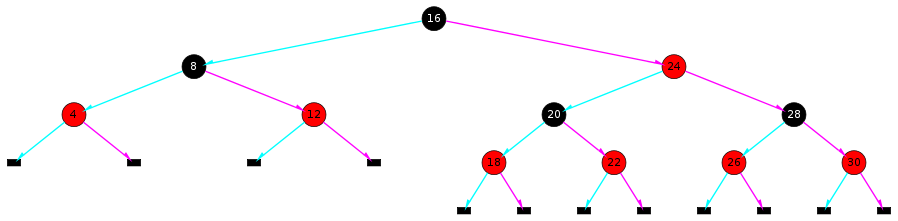Is flipping needed? If so, when should it performed? Is transformation needed? If so, when should it performed?

Go to solution of Exercise 2

### Exercise 3

Draw the process of inserting 21 into Figure 22, “Red-Black Tree sample B”.

Is flipping needed? If so, when should it performed? Is transformation needed? If so, when should it performed?

Go to solution of Exercise 3

### Exercise 4

What if the 2-3-4 root is a 4-node when doing insertion?

Go to solution of Exercise 4

## Solutions

The following are the solutions of the exercises.

### Solution to Exercise 1

Go back to Exercise 1

When going downwards, we will find a 4-node {10,12,14} and flip it: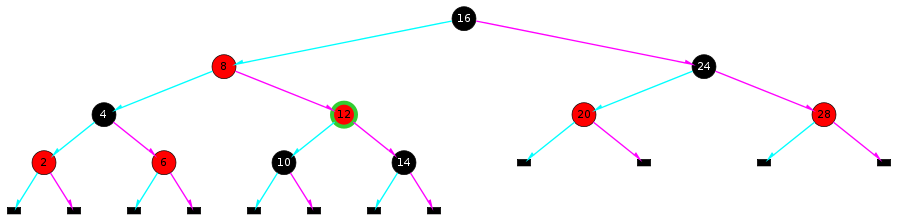Then we insert 9 as 10's left child: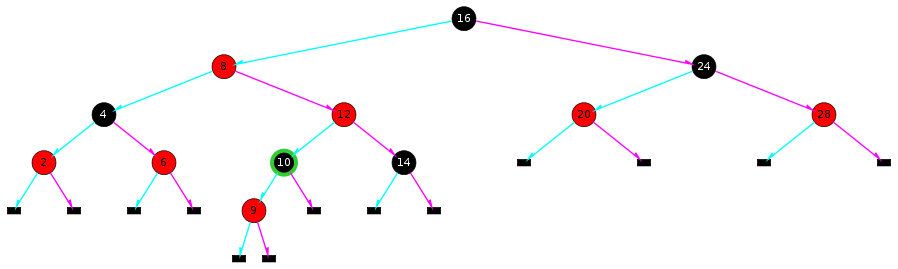This is Case.4 of The Gang of Five, so let's RotL(8):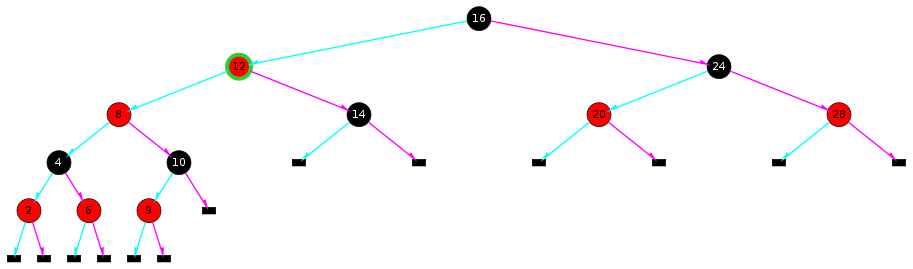Then RotR(16):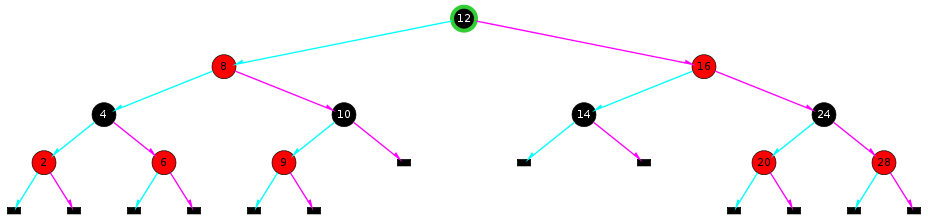Done.

Go back to Exercise 1

### Solution to Exercise 2

Go back to Exercise 2

When we go downwards, we will find a 4-node {26,28,30} and flip it: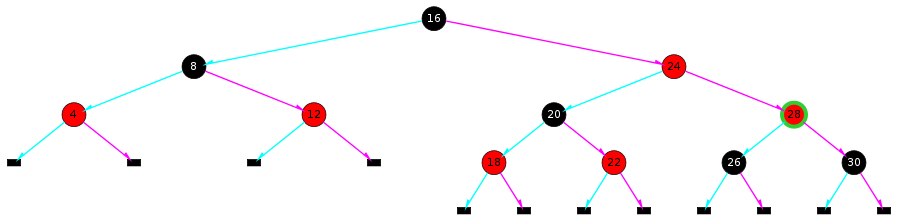Then we insert 27 as 26's right child: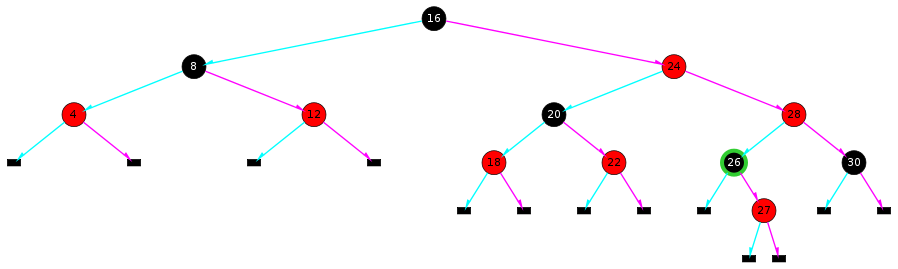When we go upwards and reach 16: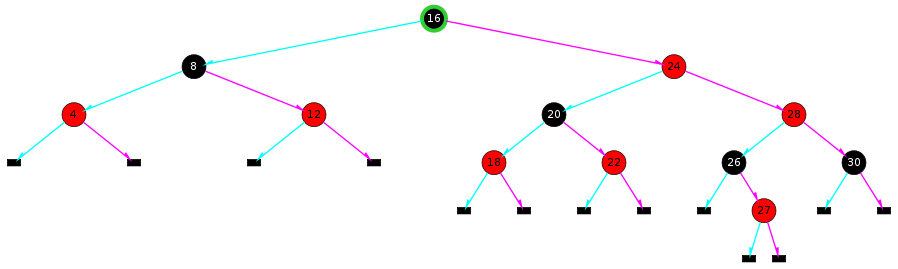We find this is Case.3 of The Gang of Five, so let's RotL(16):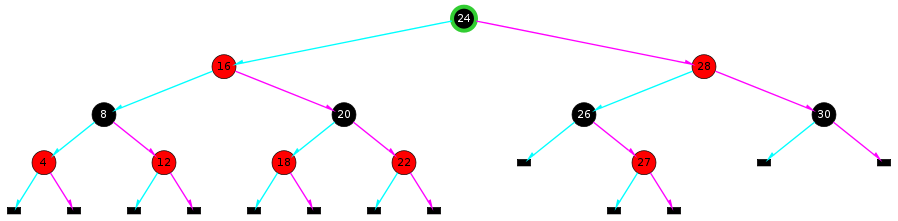Done.

Go back to Exercise 2

### Solution to Exercise 3

Go back to Exercise 3

When we go downwards, we will find a 4-node {18,20,22} and flip it: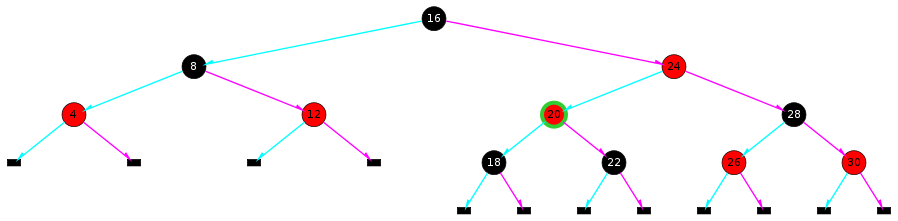Then we insert 21 as 22's left child: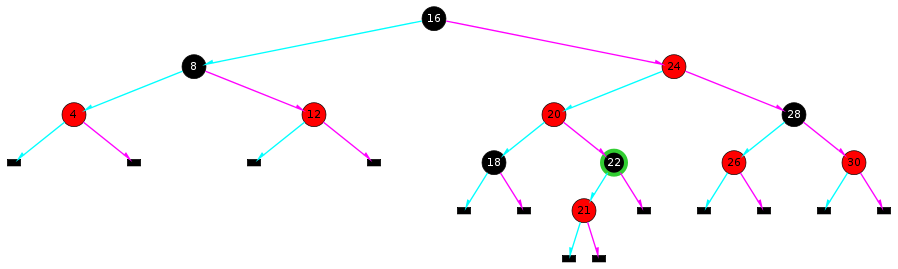When going upwards, we find this is Case.5 of The Gang of Five, so we RotR(24):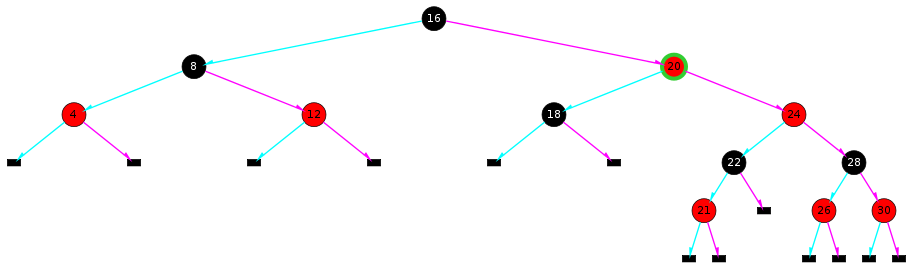Then RotL(16):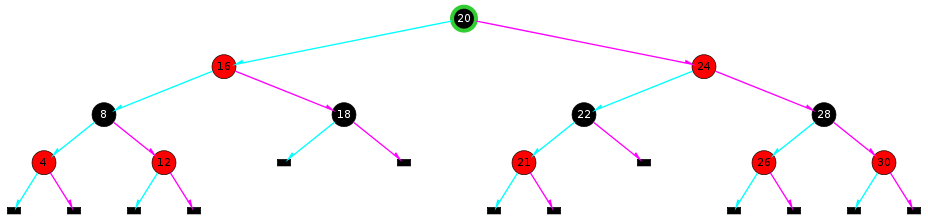Done.

Go back to Exercise 3

### Solution to Exercise 4

Go back to Exercise 4

If the 2-3-4 tree root is a 4-node, we will set the corresponding RBtree root as Red first, then set it back to Black before the end of `rb_insert()`.

Go back to Exercise 4